# Customize colormaps

This example shows how to use cmasher colormaps and also how to make a custom colormap (cmap) in Matplotlib. Colormaps are used to map from a value to a color in a chart.

```import matplotlib.pyplot as plt
import numpy as np
import pandas as pd
from matplotlib.colors import LinearSegmentedColormap
import cmasher as cmr

from mplsoccer import VerticalPitch, Sbopen
from mplsoccer.utils import FontManager

# get data
parser = Sbopen()
match_files = [19789, 19794, 19805]
df = pd.concat([parser.event(file) for file in match_files])  # 0 index is the event file

# filter chelsea pressure events
mask_chelsea_pressure = (df.team_name == 'Chelsea FCW') & (df.type_name == 'Pressure')
```

## cmasher colormaps

Cmasher colormaps are scientific colormaps that have been designed to be perceptually uniform (i.e. color changes visually look the same as the value changes) and mostly colorblind friendly. A great choice to get started and potentially more exciting than the default matplotlib choices.

Let’s first get a dictionary of all the colormaps

```cmap_dict = cmr.cm.cmap_cd
all_cmap_dict = {}
for cmap_type_key in cmap_dict:
for key, cmap in cmap_dict[cmap_type_key].items():
if key[-2:] != '_r':
all_cmap_dict[key] = cmap
```

## Cmasher kdeplot

Here’s all the cmasher colormaps plotted as a grid so you can find your favorite.

```pitch = VerticalPitch(line_color='#cfcfcf', line_zorder=2, pitch_color='#122c3d')
fig, axs = pitch.grid(nrows=11, ncols=4, space=0.1, figheight=40,
title_height=0, endnote_height=0,  # no title/ endnote
grid_width=0.9, grid_height=0.98, bottom=0.01, left=0.05)
cmap_names = list(all_cmap_dict.keys())
for idx, ax in enumerate(axs.flat):
cmap_name = f'cmr.{cmap_names[idx]}'
cmap = all_cmap_dict[cmap_names[idx]]
kdeplot = pitch.kdeplot(df.x, df.y, ax=ax, cmap=cmap, fill=True, levels=100)
ax_title = ax.set_title(cmap_name, fontsize=15)
```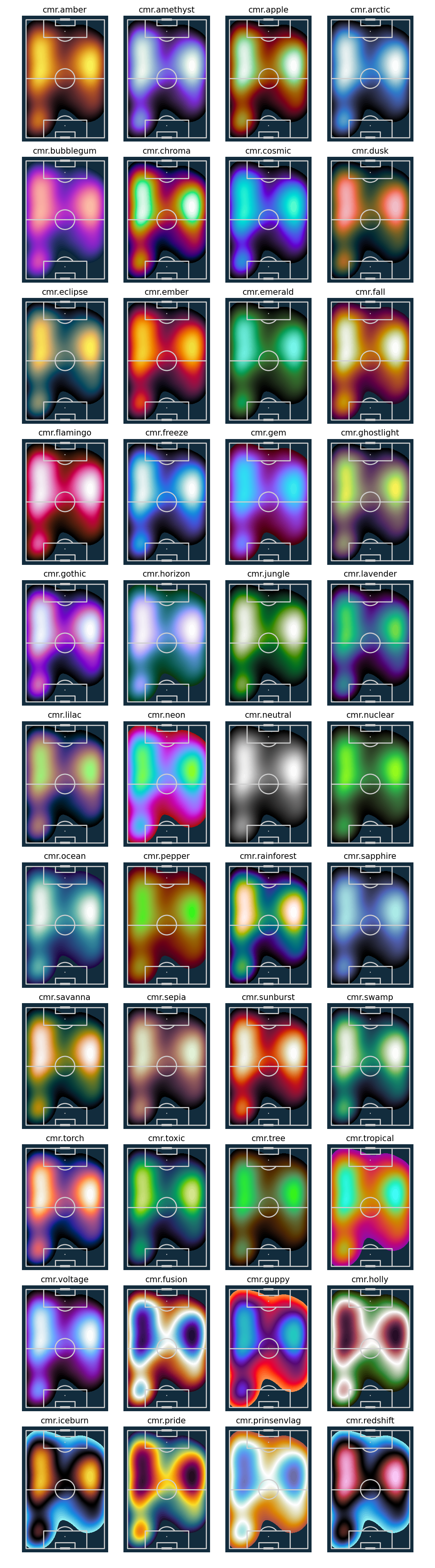```/home/docs/checkouts/readthedocs.org/user_builds/mplsoccer/conda/latest/lib/python3.11/site-packages/matplotlib/cm.py:494: FutureWarning: The 'heat' colormap was renamed to 'torch' in v1.6.1 and will be removed in v1.7.
rgba = self.cmap(x, alpha=alpha, bytes=bytes)
```

## Cmasher kdeplot

I like the look of the voltage colormap so let’s plot in against a light and dark background

You can reverse any of the colormaps, by putting _r at the end, for example cmr.arctic_r (this also applies to matplotlib cmaps).

Reversing the colormaps is sometimes helpful so the high value colors do not bleed into the background. I prefer dark to light colormaps on dark background, and light to dark colormaps on light backgrounds. I have shown this below using the same colormap in reverse.

```# dark
pitch_dark = VerticalPitch(line_color='#cfcfcf', line_zorder=2, pitch_color='#122c3d')
fig, ax = pitch_dark.draw()
kdeplot_dark = pitch_dark.kdeplot(df.x, df.y, ax=ax, cmap=cmr.voltage, fill=True, levels=100)

# light
pitch_light = VerticalPitch(line_zorder=2)
fig, ax = pitch_light.draw()
kdeplot_light = pitch_light.kdeplot(df.x, df.y, ax=ax, cmap=cmr.voltage_r, fill=True, levels=100)
```
•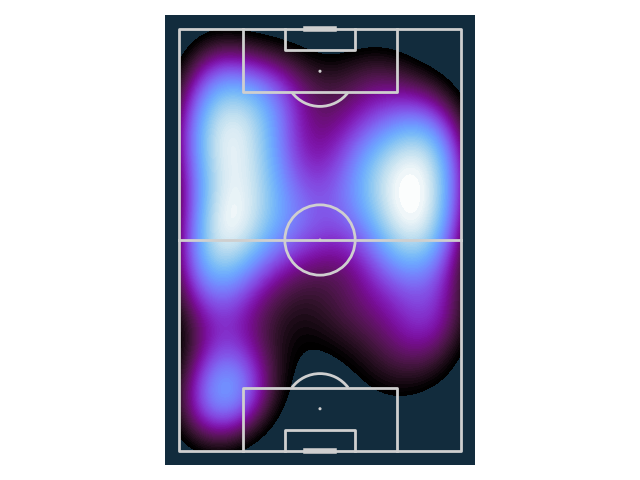•## Create colormaps using LinearSegmentedColormap

Sometimes its nice to make your own colormaps, maybe to even match team colors. In these examples we will use a list of two colors and the colormaps will linearly increase between these two colors (note you can do more such as use 3 colors).

For dark theme backgrounds, I prefer going from dark to light colors. This is so the null values which will take the dark background color are not mistaken for high values. Likewise, I prefer to go from light to dark colors when using a lighter background.

```pearl_earring_cmap = LinearSegmentedColormap.from_list("Pearl Earring - 10 colors",
['#15242e', '#4393c4'], N=10)
el_greco_violet_cmap = LinearSegmentedColormap.from_list("El Greco Violet - 10 colors",
['#332a49', '#8e78a0'], N=10)
el_greco_yellow_cmap = LinearSegmentedColormap.from_list("El Greco Yellow - 10 colors",
['#7c2e2a', '#f2dd44'], N=10)
flamingo_cmap = LinearSegmentedColormap.from_list("Flamingo - 10 colors",
['#e3aca7', '#c03a1d'], N=10)
# same color maps but with 100 colors
pearl_earring_cmap_100 = LinearSegmentedColormap.from_list("Pearl Earring - 100 colors",
['#15242e', '#4393c4'], N=100)
el_greco_violet_cmap_100 = LinearSegmentedColormap.from_list("El Greco Violet - 100 colors",
['#3b3154', '#8e78a0'], N=100)
el_greco_yellow_cmap_100 = LinearSegmentedColormap.from_list("El Greco Yellow - 100 colors",
['#7c2e2a', '#f2dd44'], N=100)
flamingo_cmap_100 = LinearSegmentedColormap.from_list("Flamingo - 100 colors",
['#e3aca7', '#c03a1d'], N=100)
```

## Show the colormaps

The below colormaps are inspired by art and nature: Girl with a Pearl by Johannes Vermeer, The Disrobing of Christ by El Greco, and flamingos.

With heatmaps and hexbins, I prefer to use fewer colors (N=10) so the values are mapped to fewer colors. While for smoother heatmaps when using kdeplot go for more colors (e.g. N=100).

```fig, axes = plt.subplots(figsize=(12, 5), nrows=8, ncols=2, constrained_layout=True)
cmaps = [pearl_earring_cmap, flamingo_cmap,
el_greco_violet_cmap, el_greco_yellow_cmap,
pearl_earring_cmap_100, flamingo_cmap_100,
el_greco_violet_cmap_100, el_greco_yellow_cmap_100]
fm = FontManager()
for i, cmap in enumerate(cmaps):
axes[i, 0].axis('off')
axes[i, 1].axis('off')
axes[i, 1].text(0, 0.5, cmap.name, va='center', fontsize=20, fontproperties=fm.prop)
```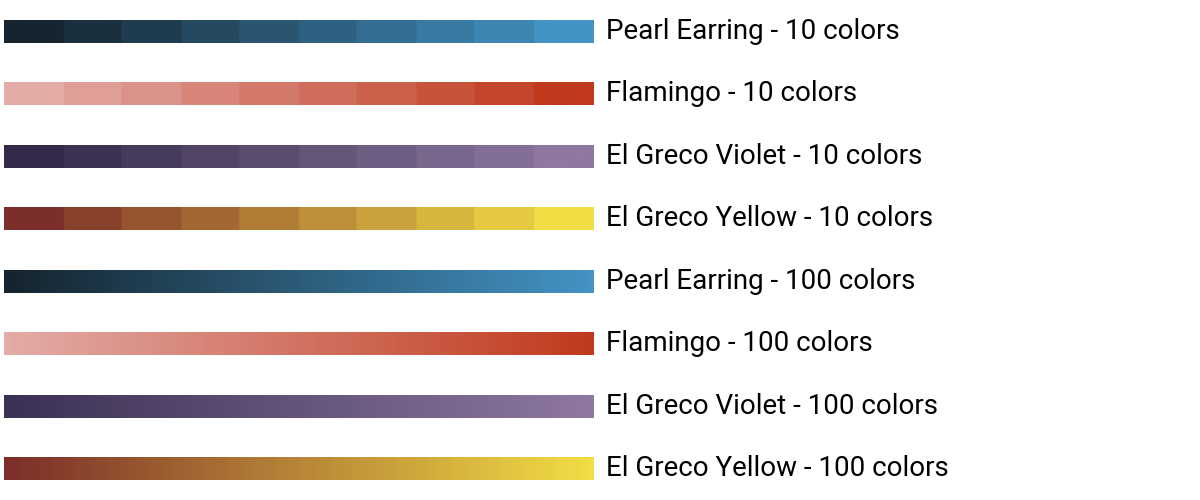Cyan colormap heatmap

```pitch = VerticalPitch(line_color='#cfcfcf', line_zorder=2, pitch_color='#122c3d')
fig, ax = pitch.draw(figsize=(4.4, 6.4))
bs = pitch.bin_statistic(df.x, df.y, bins=(12, 8))
heatmap = pitch.heatmap(bs, edgecolors='#122c3d', ax=ax, cmap=pearl_earring_cmap)
```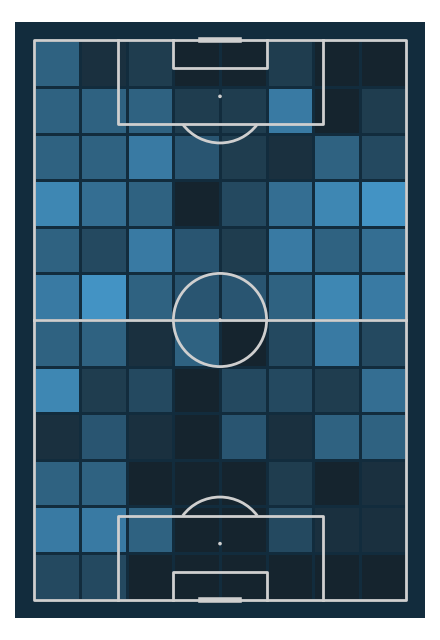Cyan colormap hexbin

```fig, ax = pitch.draw()
hexmap = pitch.hexbin(df.x, df.y, ax=ax, edgecolors='#122c3d', gridsize=(8, 8),
cmap=pearl_earring_cmap)
```Cyan colormap kdeplot

```pitch = VerticalPitch(line_color='#cfcfcf', line_zorder=2, pitch_color='#15242e')
fig, ax = pitch.draw(figsize=(4.4, 6.4))
# note use the colormap with 100 colors for a smoother finish
# sphinx_gallery_thumbnail_path = 'gallery/pitch_plots/images/sphx_glr_plot_cmap_007.png'
kdeplot = pitch.kdeplot(df.x, df.y, ax=ax, cmap=pearl_earring_cmap_100, fill=True, levels=100)
```Flamingo colormap heatmap

```pitch = VerticalPitch(line_color='#000009', line_zorder=2, pitch_color='white')
fig, ax = pitch.draw(figsize=(4.4, 6.4))
bs = pitch.bin_statistic(df.x, df.y, bins=(12, 8))
heatmap = pitch.heatmap(bs, ax=ax, edgecolors='#f4f4f4', cmap=flamingo_cmap)
```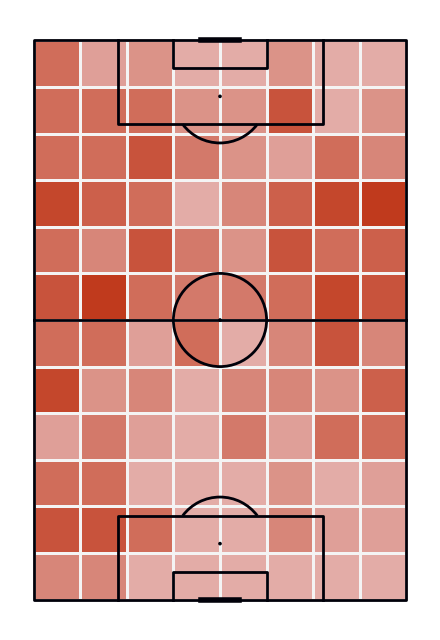Flamingo colormap hexbin

```fig, ax = pitch.draw()
hexmap = pitch.hexbin(df.x, df.y, ax=ax, edgecolors='#f4f4f4', gridsize=(8, 8), cmap=flamingo_cmap)
```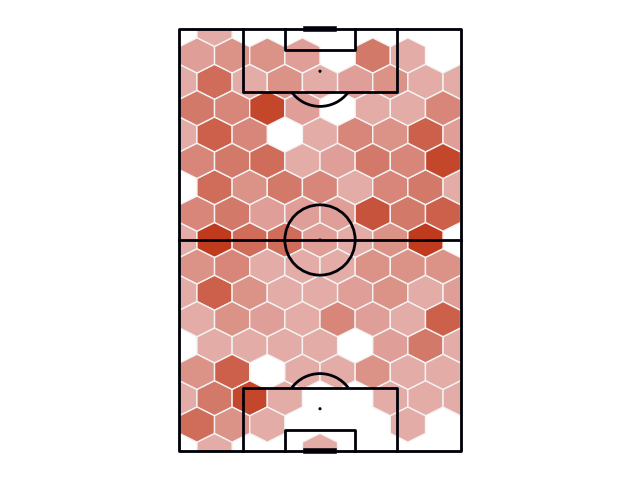Flamingo colormap kdeplot

```pitch = VerticalPitch(line_color='#000009', line_zorder=2, pitch_color='#e3aca7')
fig, ax = pitch.draw(figsize=(4.4, 6.4))
kdeplot = pitch.kdeplot(df.x, df.y, ax=ax, cmap=flamingo_cmap_100, fill=True, levels=100)
```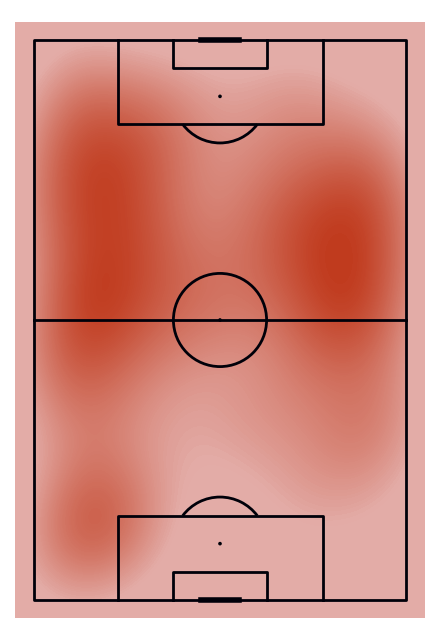Violet colormap heatmap

```pitch = VerticalPitch(line_color='#cfcfcf', line_zorder=2, pitch_color='#20143f')
fig, ax = pitch.draw(figsize=(4.4, 6.4))
bs = pitch.bin_statistic(df.x, df.y, bins=(12, 8))
heatmap = pitch.heatmap(bs, ax=ax, edgecolors='#20143f', cmap=el_greco_violet_cmap)
```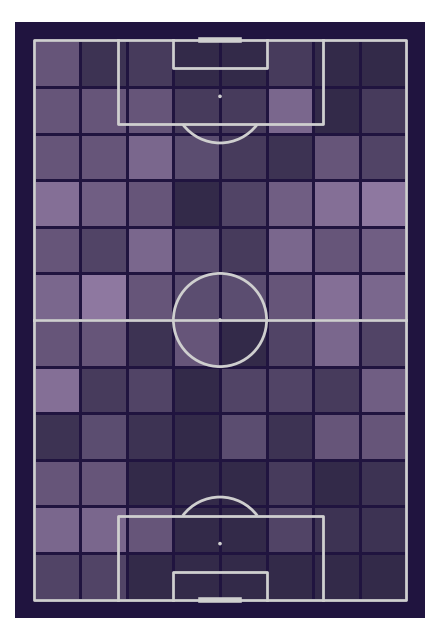Violet colormap hexbin

```fig, ax = pitch.draw()
hexbin = pitch.hexbin(df.x, df.y, ax=ax, edgecolors='#20143f',
gridsize=(8, 8), cmap=el_greco_violet_cmap)
```Violet colormap kdeplot

```pitch = VerticalPitch(line_color='#cfcfcf', line_zorder=2, pitch_color='#332a49')
fig, ax = pitch.draw(figsize=(4.4, 6.4))
kdeplot = pitch.kdeplot(df.x, df.y, ax=ax, cmap=el_greco_violet_cmap_100, fill=True, levels=100)
```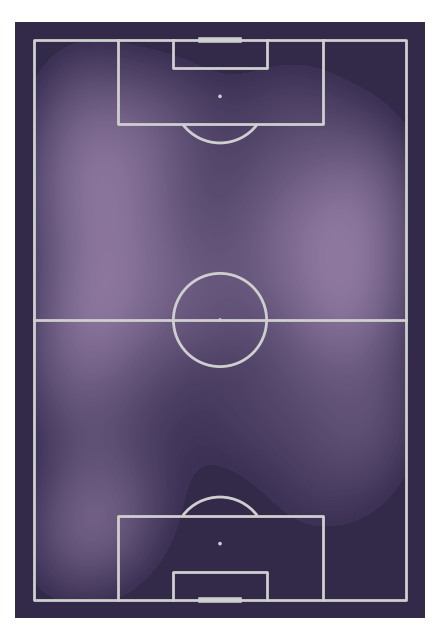Yellow colormap heatmap

```pitch = VerticalPitch(line_color='#cfcfcf', line_zorder=2, pitch_color='#471c15')
fig, ax = pitch.draw(figsize=(4.4, 6.4))
bs = pitch.bin_statistic(df.x, df.y, bins=(12, 8))
heatmap = pitch.heatmap(bs, ax=ax, edgecolors='#471c15', cmap=el_greco_yellow_cmap)
```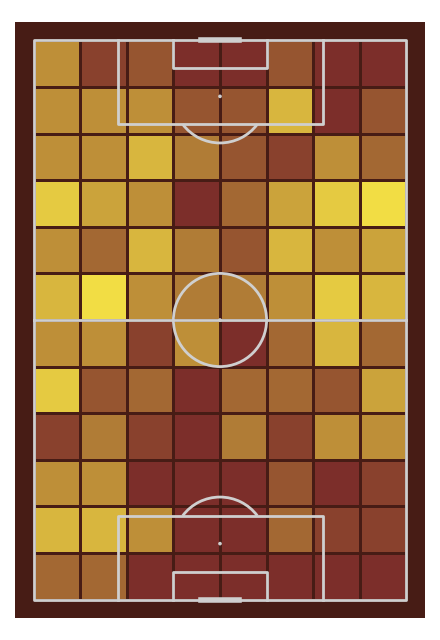Yellow colormap hexbin

```fig, ax = pitch.draw()
hexbin = pitch.hexbin(df.x, df.y, ax=ax, edgecolors='#443d07',
gridsize=(8, 8), cmap=el_greco_yellow_cmap)
```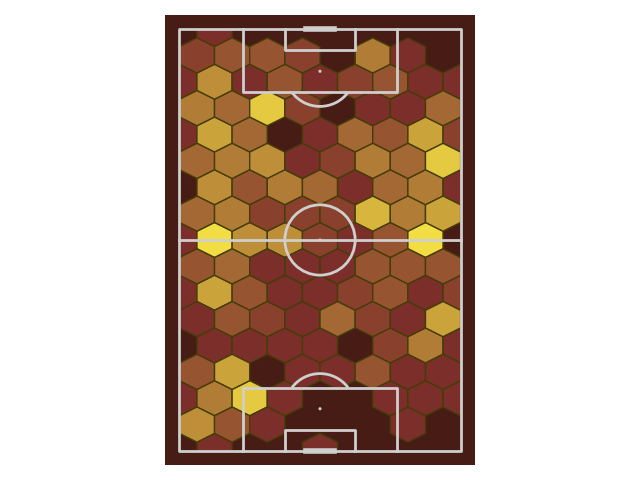Yellow colormap kdeplot

```pitch = VerticalPitch(line_color='#cfcfcf', line_zorder=2, pitch_color='#7c2e2a')
fig, ax = pitch.draw(figsize=(4.4, 6.4))
kdeplot = pitch.kdeplot(df.x, df.y, ax=ax, cmap=el_greco_yellow_cmap_100, fill=True, levels=100)

plt.show()  # If you are using a Jupyter notebook you do not need this line
```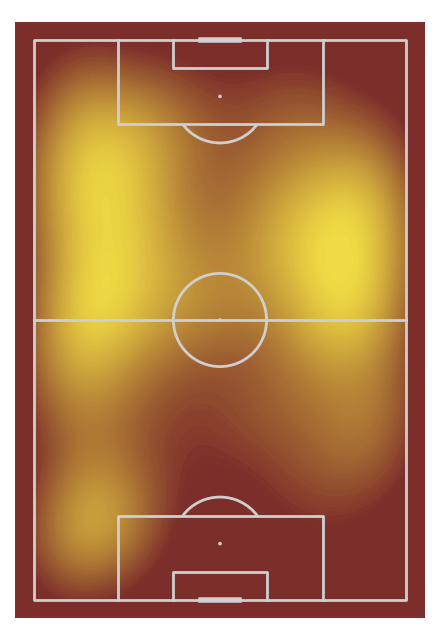Total running time of the script: ( 0 minutes 30.813 seconds)

Gallery generated by Sphinx-Gallery